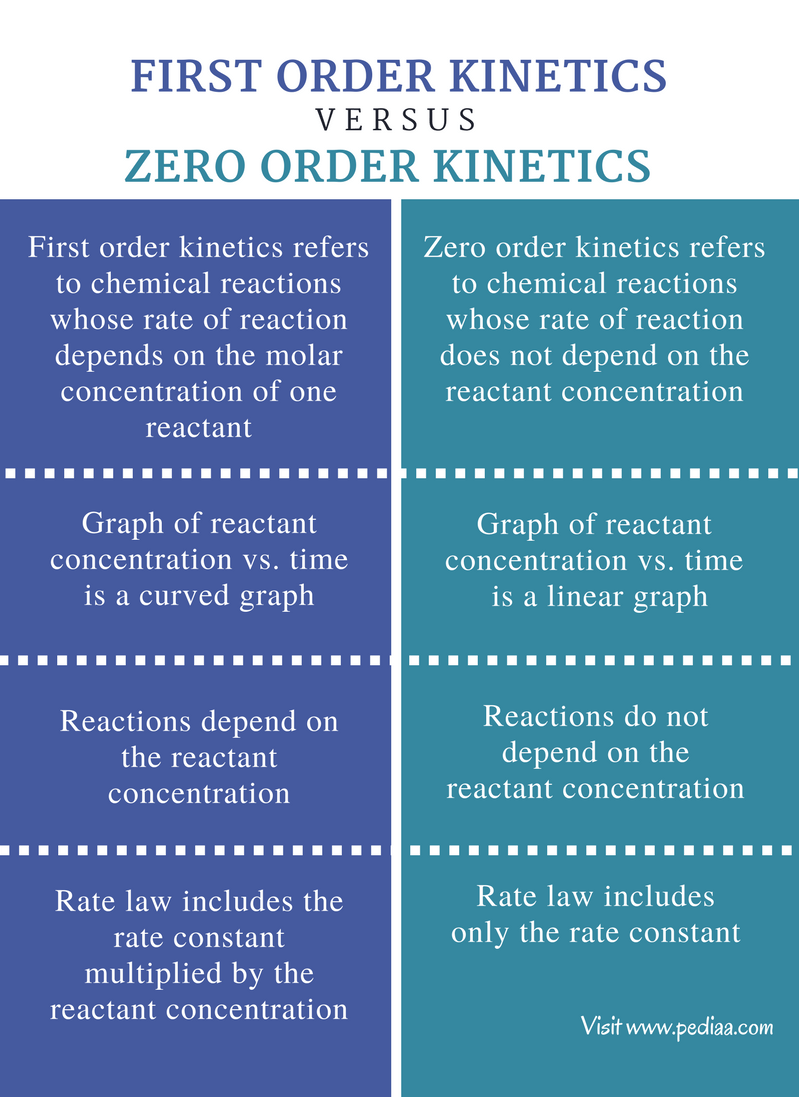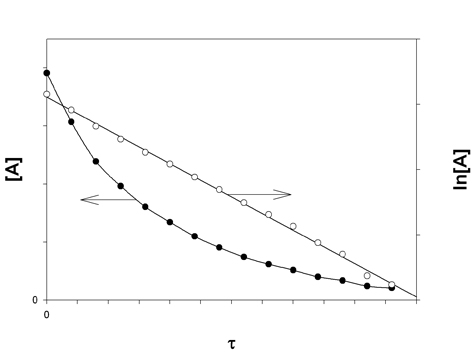# Difference Between First Order and Zero Order Kinetics

## Main Difference – First Order vs Zero Order Kinetics

Chemical kinetics describes the rates of chemical reactions. The concept of chemical kinetics was first developed by the law of mass action. The law of mass action describes that the speed of a chemical reaction is proportional to the mass of reactants. According to chemical kinetics, reactions can be categorized as zero order reactions, first order reactions, and second order reaction.  The main difference between first order and zero order kinetics is that the rate of first order kinetics depends on the concentration of one reactant whereas the rate of zero order kinetics does not depend on the concentration of reactants.

### Key Areas Covered

1. What is First Order Kinetics
– Definition, Properties, Examples
2. What is Zero Order Kinetics
– Definition, Properties, Examples
3. What is the Difference Between First Order and Zero Order Kinetics
– Comparison of Key Differences

Key Terms: Concentration, First Order Kinetics, Kinetics, Law Of Mass Action, Rate Constant, Rate Law, Rate of Reaction, Zero Order Kinetics## What is First Order Kinetics

First order kinetics refers to chemical reactions whose rate of reaction depends on the molar concentration of one reactant. The rate of the reaction is proportional to the concentration of one reactant. There can be many other reactants taking part in the chemical reaction, but only one reactant will determine the rate of the reaction. Therefore, the other reactants are known to be in zero order with respect to this particular reaction.

For example, let’s consider the decomposition of dinitrogen pentoxide (N2O5). This is a unimolecular reaction. That means, this reaction is composed of only one reactant. The rate of the reaction can be given as below.

2N2O5(g)     →     4NO2(g)    +    O2(g)

Rate =  k[N2O5(g)]m

k is the rate constant and [N2O5(g)] is the concentration of N2O5(g). The letter “m” gives the order of the reaction regarding the concentration of N2O5(g). The above equation is known as the rate law and for the above equation, m=1. Then the rate of the reaction can be given as below.

Rate = k[N2O5(g]

The value of the m can be obtained experimentally. Here, the value would always be one. That indicates the decomposition of N2O5(g) is a first order reaction. In addition, the order of the reaction may or may not equal to the stoichiometric coefficient of the reactants.  In the above example, the order of the reaction is 1 although the stoichiometric coefficient is 2. The rate of the first order reaction can be shown in a graph as below.Figure 1: Graph of first order kinetics

In the above diagram, the graph with dark points is the graph of reactant concentration vs. reaction time. It is a curved graph which indicates that the rate of the reaction is changed with concentration of the reactant. The graph with white colored points shows the graph of ln[reactant concentration] vs. reaction time. It is a linear graph.

## What is Zero Order Kinetics

Zero order kinetics refers to chemical reactions whose rate of the reaction does not depend on the reactant concentration. In other words, the concentration of reactants does not affect the rate of the reaction. Therefore, as long as the temperature is constant, the reaction rate would be constant in zero order kinetics.

No matter what reactants are present and to what extent their concentrations have been changed, the rate of the reaction would remain the same. Therefore, the rate of the reaction is given as,

Rate =  k

Where, k is the rate constant.

A good example for zero order reactions is decomposition of nitrous oxide in the presence of platinum as catalyst.

2N2O(g)    →     2N2(g)      +     O2(g)

The rate of this reaction is equal to the rate constant. Hence, the rate of the reaction can be given as below.

Rate = k[N2O(g)]0Figure 2: The graph of reactant concentration vs. reaction time

The above graph shows the variation of the concentration of reactants with the reaction time for zero order kinetics. It is a linear graph.

## Difference Between First Order and Zero Order Kinetics

### Definition

First Order Kinetics: First order kinetics refers to chemical reactions whose rate of reaction depends on the molar concentration of one reactant.

Zero Order Kinetics: Zero order kinetics refers to chemical reactions whose rate of the reaction does not depend on the reactant concentration.

### Graph of Reactant Concentration vs. Time

First Order Kinetics: The graph of reactant concentration vs. time for first order kinetics is a curved graph.

Zero Order Kinetics: The graph of reactant concentration vs. time for zero order kinetics is a linear graph.

### Reactant Concentration

First Order Kinetics: The first order kinetic reactions depend on the reactant concentration.

Zero Order Kinetics: The zero order kinetic reactions do not depend on the reactant concentration.

### Rate Law

First Order Kinetics: The rate law of the first order kinetic reactions includes the rate constant multiplied by the reactant concentration.

Zero Order Kinetics: The rate law of the zero order kinetic reactions includes only the rate constant.

### Conclusion

The rate law or the rate equation gives the most important details about the chemical kinetics of systems. It describes the rate of a particular reaction regarding the reactant concentration and the rate constant at a constant temperature. According to the kinetics of chemical reactions, there are three major types of reactions. They are zero order reactions, first order, reactions and second order reactions. These reactions differ from each other according to the order of the reaction with respect to the reactants present in a particular system.

##### References:

1. “First-Order Reactions.” Chemistry LibreTexts. Libretexts, 04 July 2017. Web. Available here. 14 July 2017.
2. “Zero-Order Reactions.” Chemistry LibreTexts. Libretexts, 21 July 2016. Web. Available here. 14 July 2017.

##### Image Courtesy:

1. “First Order” By Flanker – Own work (CC BY-SA 4.0) via Commons Wikimedia